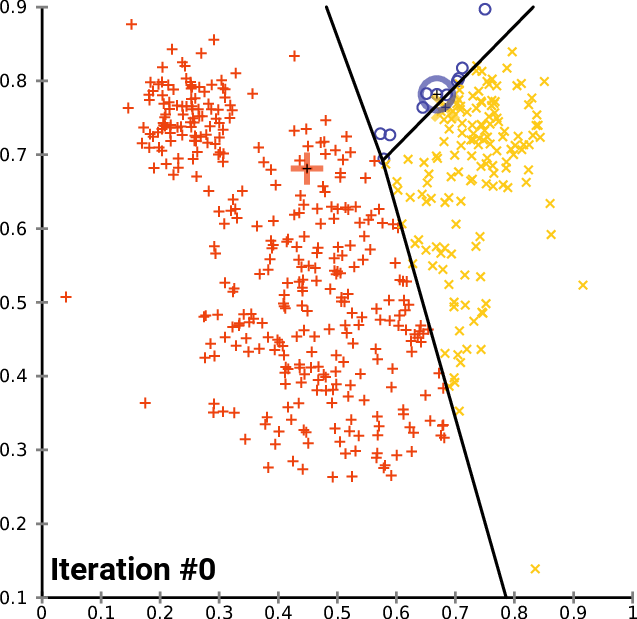# The basis

• K-means clustering is an unsupervised learning method.
• The aim is to find clusters and the CentroIDs that can potentially identify the
• What is a cluster? a set of data points grouped in the same category.
• What is a CentroID? center or average of a given cluster.
• What is “k”? the number of CentroIDs

# Typical questions you will face

• The number k is not always known upfront.
• First look for the number of CentroIDs, then find the k-values (separate the 2 problems).
• Is our data “clusterable” or it is homogeneous?
• How can I determine if the dataset can be clustered?
• Can we measure its clustering tendency?

#A real situation: identification of geographical clusters

You can create a K-means algorithm, where the distance is used to know the similarities or dissimilarities. Any pointers how a properties of observations are mapped so that you can decide the groups based on the K-means or hierarchical clustering.

Since k-means tries to group based solely on euclidean distance between objects you will get back clusters of locations that are close to each other.

To find the optimal number of clusters you can try making an ‘elbow’ plot of the within group sum of square distance.

http://nbviewer.jupyter.org/github/nborwankar/LearnDataScience/blob/master/notebooks/D3.%20K-Means%20Clustering%20Analysis.ipynb.